# C Program to Determine Whether a Number is Prime or Not

In this post, we will learn how to create a program in C that will check whether the given number (as given by the user at run-time) is a prime number or not. We will also learn how to print all prime and composite numbers present between any two numbers, say 1 and 20; that is, all prime and composite numbers between 1 and 20.

At last, we have created a program that will ask the user to enter any two numbers to display all the prime and composite numbers present between the two numbers. Let's first take a look at the program that will check whether the number entered by the user is prime or not.

### How to Find the Prime Number

If a number is divisible by any number from 2 to one less than the number (i.e., n-1), let's suppose that the user has supplied a number, say 37, as input. If 37 is divisible by any number from 2 to 36, then the number is not a prime number; otherwise, it will be a prime number.

Note: A prime number is a number that cannot be divided by any number except 1 and the number itself.

## Check for the prime number in C

To check whether the input number is a prime number or not in C programming, you have to ask the user to enter a number and start checking for a prime number, as shown in the program given below.

```#include<stdio.h>
#include<conio.h>
int main()
{
int num, i, count=0;
printf("Enter a number: ");
scanf("%d", &num);
for(i=2; i<num; i++)
{
if(num%i == 0)
{
count++;
break;
}
}
if(count==0)
printf("\nIt's a prime number");
else
printf("\nIt's not a prime number");
getch();
return 0;
}```

As the above program was written in the Code::Blocks IDE, here is the sample after a successful build and run. This is the first snapshot of the sample run: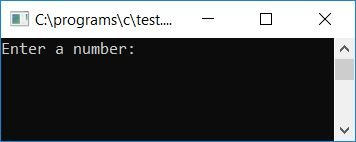Supply any number, say 37, and press the ENTER key to check whether it is a Prime number or not. Here is the second snapshot of the sample run: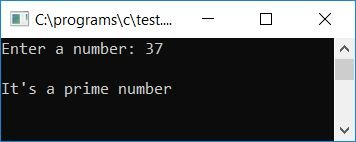#### Program Explained

• Receive any number as input, say 37.
• Make a for loop that goes from 2 to one less than the number, so 37-1 or 36.
• Inside the for loop, check whether the current value of the loop variable, say i, divides the number, say 37 (given by the user at run-time), without leaving any remainder or not.
• If it divides, then program flow goes inside the if block to increment the value of any variable, say count here, and then use the break keyword to exit from the loop. As if any number except 1 and the number itself divided the number, that meant the number was not a prime number.
• Never forget to initialize 0 to the count variable as its initial value at the start of the program.
• Now after exiting from the loop, whether using the break keyword or normally, check whether the count variable holds its original value or not, if it holds (that is, 0), it means the program flow never goes inside the if block, and it means any number from 2 to 36 does not completely divide the number, therefore the given number is a prime number.
• Otherwise, if the count variable holds a value other than 0, then it means that the given number is not a prime number.

### Print all prime and composite numbers in C

Now let's create a program that will find and print all prime and composite numbers present in the range of 1 to 20.

```#include<stdio.h>
#include<conio.h>
int main()
{
int i, j, count=0;
printf("\t\tBetween 1 to 20:\n\n");
printf("Prime Numbers\t\tComposite Numbers\n");
for(i=1; i<=20; i++)
{
for(j=2; j<i; j++)
{
if(i%j == 0)
{
count++;
break;
}
}
if(count==0)
printf("%d", i);
else
printf("\t\t\t%d", i);
count=0;
printf("\n");
}
getch();
return 0;
}
```

After a successful build and run, here is the sample run. This is the first screenshot: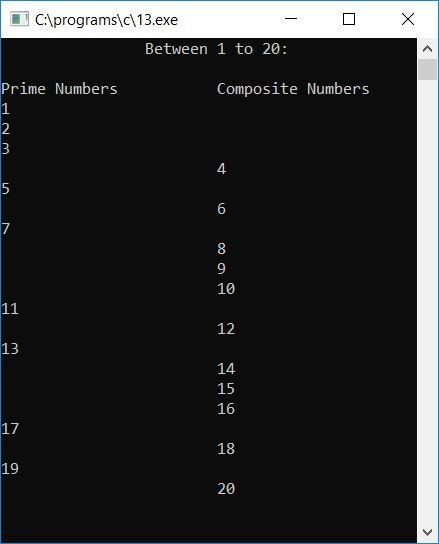#### Program Explained

• Create a for loop that runs from 1 to 20.
• For each and every value of the loop variable, say i (1, 2, 3, 4, ... 20,) check whether it is a prime or not.
• We've made two columns here: one for prime numbers and another for composite numbers.
• If the number is found to be a prime number, then print it without adding any horizontal tabs (\t).
• And if the number is found as a composite number, then print it after two or three horizontal tabs to show it in the second column.
• Now to the point, inside the for loop, we have created another for loop with another loop variable, say j, and here we have run the loop from 2 to one less than the outer loop variable's current value (i-1). That is, if the current value of i is 17, then check whether any number from 2 to 16 divides the number 17 totally (without leaving any remainder) or not; if it divides, then increment the count variable and use the break keyword to exit from the inner for loop.
• Now check whether count holds the original or initial value or not. If it holds, then print the number as a prime number in the first column, that is, without adding a horizontal tab before the number.
• And if count is not 0, then print the number as a composite number in the second column, after some horizontal tabs.
• Never forget to initialize 0 again to the count variable before going back to the first for loop to again check and do the same steps as told above.
• In this way, all the prime and composite numbers get printed as output.

Let's create the same program, but this time the program given below displays the prime number in one line and the composite number in the second line. This program will not display the prime and composite numbers in table form. Here is the program:

```#include<stdio.h>
#include<conio.h>
int main()
{
int i, j, count=0, arr, k=0;
printf("\t\tBetween 1 to 20:\n\n");
printf("Prime Numbers: ");
for(i=1; i<=20; i++)
{
for(j=2; j<i; j++)
{
if(i%j == 0)
{
count++;
break;
}
}
if(count==0)
printf("%d ", i);
else
{
arr[k] = i;
k++;
}
count=0;
}
printf("\nComposite Numbers: ");
for(i=0; i<k; i++)
printf("%d ", arr[i]);
getch();
return 0;
}```

Here is the sample: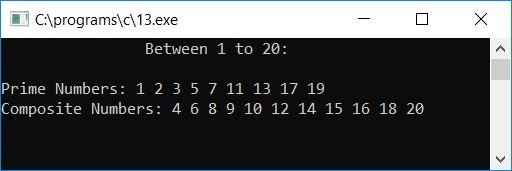In the above program, we have checked whether the given number is a prime number or not, as told in the above steps; if it is, then print it out; otherwise, initialize the current value to any array. This array's elements will be all the composite numbers between 1 and 20.

### Allow the user to specify a range

The question is, "Write a program in C to print all composite and prime numbers between the given range:

```#include<stdio.h>
#include<conio.h>
int main()
{
int i, j, count=0, arr, k=0, rangeStart, rangeEnd;
printf("Enter Starting Number: ");
scanf("%d", &rangeStart);
printf("Enter Ending Number: ");
scanf("%d", &rangeEnd);
printf("\t\tBetween %d to %d:\n\n", rangeStart, rangeEnd);
printf("Prime Numbers: ");
for(i=rangeStart; i<=rangeEnd; i++)
{
for(j=2; j<i; j++)
{
if(i%j == 0)
{
count++;
break;
}
}
if(count==0)
printf("%d ", i);
else
{
arr[k] = i;
k++;
}
count=0;
}
printf("\nComposite Numbers: ");
for(i=0; i<k; i++)
printf("%d ", arr[i]);
getch();
return 0;
}```

The snapshot given below is a sample run of the above program: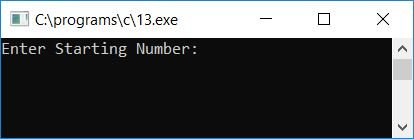Now enter the range. Let's suppose the user has supplied 10 as the starting number and 40 as the ending number. Therefore, here is the output you will see on your output screen after pressing the ENTER key: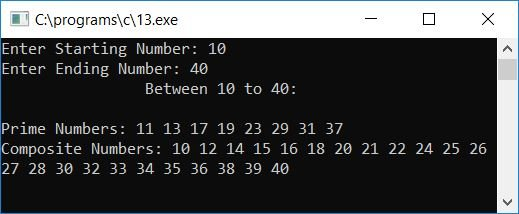#### The same program in different languages

C Quiz

« Previous Program Next Program »